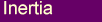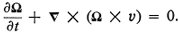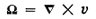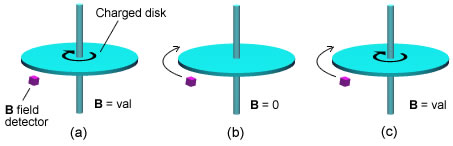##### 3 - MagnetismHere we will examine the nature of magnetism and its formation within the context of an aether model.

#### Magnetism as the Flow of Aether

It is well known that Maxwell’s equations have many similarities to the hydrodynamic equations for a fluid. This is not surprising given that the original Maxwell’s equations were derived on the basis of a fluidic medium (aether) .
It is worthwhile looking at these similarities more closely.

If we consider the general equations for fluid flow where we are only interested in describing the velocity, we get the following equations :where v is the velocity of the fluidis the vorticity, or circulation of the fluidis in fact a vector that points in the direction perpendicular to the circulation and whose magnitude is proportional to the amount of circulation, Fig 3.1(a).

If we now look at the Maxwell’s equations that describe the magnetic field we have, for the case where there are no changing electric fields, the following:where B is the magnetic field
j is the electric current

We can immediately see the similarities between these and the last two equations above if we equate the velocity v with B and vorticitywith the current j.
This suggests that, at least under certain conditions, the magnetic field represents the velocity of the aether as projected onto 3D space. That is, as there is a 4D component to the circulation of the aether (see next item) the aether particles would not move parallel to the 3D velocity vector above but would move in and out of the 4th dimension, with the 3D projected velocity being represented by v.
We can get a better idea of what is involved by considering a normal 3D vortex such as one observes in water draining from a bathtub. If we then imagine a 2D plane (x, y) at right angles to the vortex axis (z), the water molecules will follow a spiral path which appears circular when projected onto the 2D plane. However 2D flatlanders living on the plane would not see the water molecules going in a circle, rather the molecules will appear briefly as they cross the 2D plane before disappearing again from their awareness.
We would expect something similar to occur when going from 3D to 4D space.Fig 3.1 Comparison between magnetic field and fluid flow.

We can apply this idea to the case of a current carrying wire, Fig 3.1(b).

We know from experiments that the magnetic field around a current carrying wire falls off as 1/r, where r is the distance from the wire, and is proportional to the current.
This would imply that the projected 3D velocity of the aether also falls off as 1/r with a magnitude that is proportional to the current.

Based on the previous discussion about the similarity of the current and the vorticity this would suggest that the moving charges that form the current somehow create a preferential rotation of the aether around the wire.
Given that electric currents are generated by moving charges it remains to be explained how a moving charge can create a circulating aether perpendicular to the direction of motion.

#### Moving Charges and Magnetic Fields

As detailed in the previous section, a charge is considered to be a vortex of aether entering the 4th dimension, Fig 2.1 .

Lets consider the case when the charge moves in 4D.
One might expect that the aether vortex, whose axis is perpendicular to 3D space - Fig 2.1 , would lean over behind the direction in which the charge is being pulled, Fig 3.2.
This of course is a much simplified picture of a 4D vortex.
We would then have a net component of the vortex spin around the direction of motion where the 4D axis meets 3D space.Fig 3.2 Electron vortex motion in 4D.

In other words the aether flow from a charge would not be spherically symmetric but would show a preferential rotational movement around the direction of motion.
The magnitude of that preferential spin would depend on the speed of that motion relative to the aether background. The faster the charge moves the more the vortex would lean over in 4D and the greater would be the component of spin projected onto 3D.
Motions in 4D are difficult to picture but it does make intuitive sense.

This preferential rotation of the aether around a moving charge would produce what we observe as the magnetic field, when all the individual charge contributions are summed.

This picture also neatly accounts for the fact that when a charge reverses direction of motion the magnetic field rotates in the opposite sense. That is, the vortex leans over in the opposite direction and therefore the projected spin goes from clockwise to anticlockwise or vice versa.

The idea that a charge corresponds to an aether vortex and the magnetic field to a moving aether 'fluid' is further supported by the work of R. M. Kiehn who has shown that there exists a direct mapping between the Schroedinger wave equation for a charged particle in a magnetic field and the Navier-Stokes fluid dynamic equations for vortex motion in a compressible viscous fluid. See the Atoms&QM page for more on this.

#### Aether, Magnetism and Relativity

Note that the above implies that the magnetic field from a charge depends on the speed of the charge relative to the aether background and not relative to the observer as standard theory suggests.

The idea that the magnetic field should depend on how fast a charge is moving relative to the observer leads to a number of conceptual difficulties and paradoxes. Where is the actual field in space if it depends on the observer?
By assuming that the magnetic field is dependent on the speed of the charges relative to the aether a lot of the complexity simply disappears.
There is a logical requirement (Lorentz invariance) that the forces between objects, such as the force between two current carrying wires, should not depend on what the observer is doing.
In the aether model this condition will be satisfied in a natural way because the forces surrounding moving charges will not depend on the speed of the charges relative to the observer but relative to the aether.
Lets see if this is consistent with observation.

We can consider the situation of two parallel current carrying wires that attract each other by virtue of their magnetic fields, Fig 3.3.Fig 3.3  The force F between two current carrying wires.

One might ask what happens if in the above example we move both the wires in the opposite direction to the movement of the conduction electrons by an amount equal to the drift velocity of the electrons.
In other words, the wires are moved in such a way as to make the conduction electrons stationary, on average, with respect to the aether.
Shouldn’t this make the magnetic field disappear, contrary to experience?

On closer examination we find that moving the wires in this way causes the positive charges of the atoms to move relative to the aether by an amount equal and opposite to the drift velocity of the electrons (Vd in Fig 3.3).
This movement of the positive charges relative to the aether will create exactly the same magnetic field as the conduction electrons do for the stationary wire case.
In general, moving the wires at any speed will create a magnetic field that is the difference between the positive and negative charge contributions.
This means that the net magnetic field will always be proportional to the average drift velocity of the conduction electrons relative to the wire no matter what the speed of the wires relative to the aether.

It is not too difficult to see that any neutral object, carrying any current whatever, will behave in exactly the same way in both the aether model and the standard theory.
Things get more interesting however when we have objects with a net charge moving through the aether.

It is worth noting that the aether theory does not eliminate relativistic type effects. When two objects move relative to one another relativistic effects will come into play because of the finite speed of the forces, which is what relativity takes into account.

#### Differences Between the Aether Model and Standard Theory

It follows from the logic in the previous section that an object with a net charge moving relative to the aether should produce a magnetic field even for an observer traveling with the object.
Here we have a distinct difference between our aether model and standard theory.

One might ask if this difference really exists how is it possible that this discrepancy to standard theory has not been noticed up to now.
We believe there are two reasons for this.

Firstly, any experiments done with moving charges where the observer is stationary with respect to the surface of the earth, will produce the same results in the two cases.
This comes about because the aether is assumed to be stationary with respect to the surface of the earth so that the speed of the charges relative to the aether will be the same as the speed relative to the observer.

The second reason is that even when the observer is moving with the charges the magnetic field predicted by the aether model is quite small for most cases that we encounter.
To see this we consider an extreme example of a 1m diameter conducting sphere charged to 1 million Volts. By standard electrostatic equations we can calculate the charge on the sphere to be around 5x10-5 Coulombs.
If we now moved the sphere at 100km/hr along the surface of the earth it would equate to a current of around 0.015 Amps.
In the aether theory this would produce a magnetic field of around 2x10-9 Teslas near the surface of the sphere, or around 10-5 the strength of the earth’s magnetic field.
Quite easy to miss if one were not specifically looking for it!

There is however another situation where we might expect to see a much greater effect.
All things being equal, the electrostatic forces between two charged bodies are much greater than the equivalent magnetic forces at normal charge velocities.
One would therefore expect that the force between two charged bodies would be affected by the movement through the aether and might be detected under normal conditions.

There is in fact some experimental support for this idea.
Charles Morton has reported observing a variation of the force between strongly charged bodies when they are set in motion as compared to the stationary case, even for small speeds .
The force was also found to be different in front compared to the rear of the moving charges.

This experiment, if it can be replicated, has important implications to the current understanding of how things work.
According to standard theory the forces between charged bodies, not in motion relative to one another, should be the same in all inertial reference frames (frames moving at constant speeds).
The 'Morton Effect' would point to, among other things, a universal reference frame or aether.

#### Further Experimental Support for the Aether Model

If we follow further the consequences of the aether model it predicts that an observer (detector) moving relative to a charge that is stationary with respect to the aether will not see the usual magnetic field.
This is in disagreement with standard theory which predicts a B field whenever an observer moves relative to charge.
Stefan Marinov carried out an experiment that is relevant to this prediction. He used a Hall effect detector to measure the magnetic field produced by a spinning charged disk, Fig 3.4, a variation on the Rowland experiment.Fig 3.4  The Marinov experiment, (a) detector is stationary and disk spins, (b) detector rotates
and disk is stationary, (c) detector and disk spin together.

He reported the following results for 3 variations of the experiment:
(a) The detector is stationary and charged disk spins. This produced a B field.
(b) The detector rotates but the charged disk is stationary. This did not produced a B field.
(c) Both the detector and disk spin together producing the same reading as in (a).

We will analyze these findings with the standard theory and the aether model.<

Standard Theory
(a) There is relative movement between the detector and the charges so we expect a B field.
This agrees with observation.
(b) There is relative movement between the detector and the charges so we expect a B field.
This does not agree with observation.
(c) The detector is completely stationary relative to the charges so we would not expect a B
field to be registered.
This does not agree with observation.

Aether Model
(a) Charges move relative to the aether so we would expect a B field.
This agrees with observation.
(b) Charges do not move relative to the aether so we would not expect a B field.
This agrees with observation.
(c) Charges move relative to the aether so we would expect a B field even though there is no
movement of the detector relative to the charges.
This agrees with observation.

As we can see the results are in disagreement with standard theory but are completely consistent with the aether model.

#### References

 Sir Edmund Whittaker, “History of the Theories of Aether and Electricity”, Nelson LTD, N.Y. 1953
 Feynman RP, Leighton, RB, Sands M, “The Feynman Lectures on Physics”, Addison-Wesley, Vol II, Sec 40-42
 Charles R. Morton, "Velocity Alters Electric Field", www.amasci.com/freenrg/morton1.html